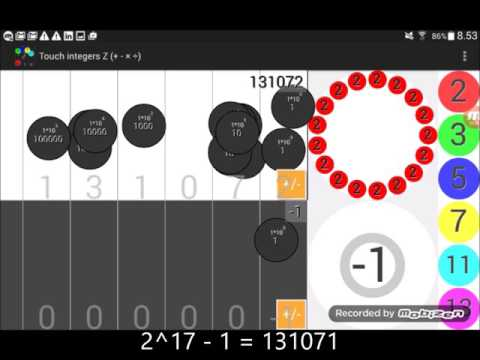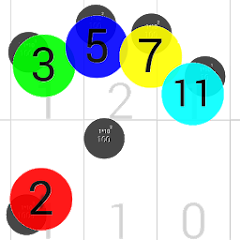# Touch Integers ℤ (+ - × ÷)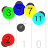1K+Everyone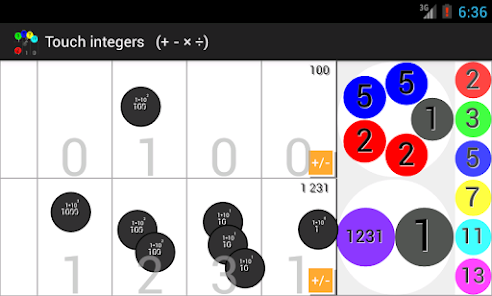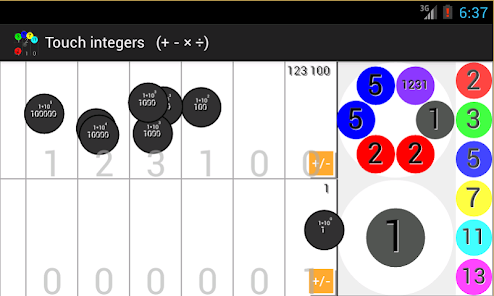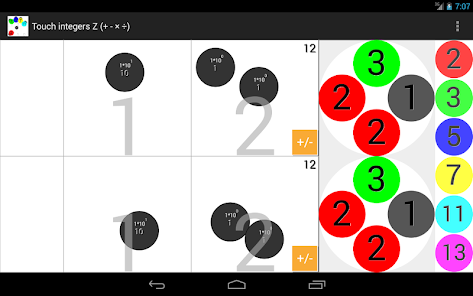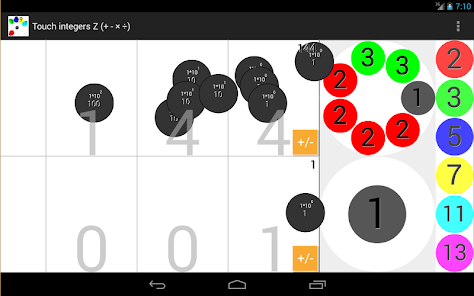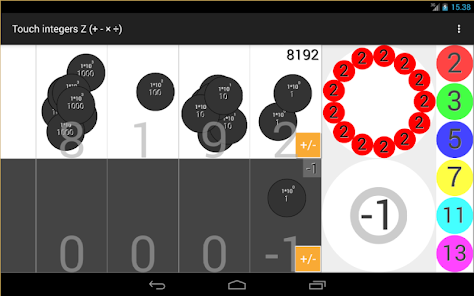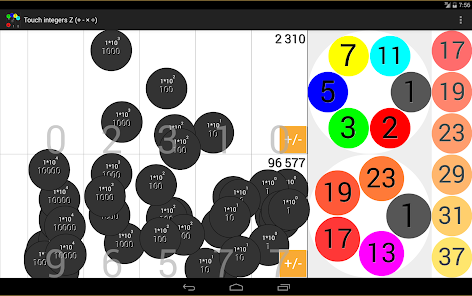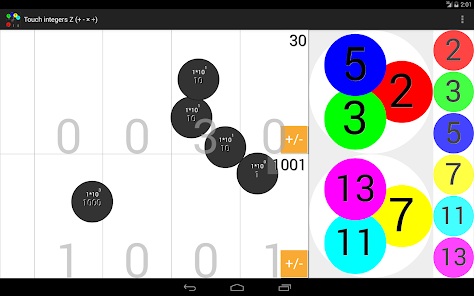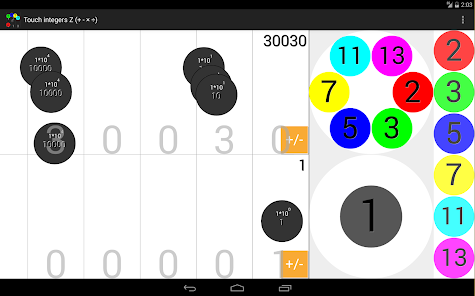The fundamental theorem of arithmetic in practice:
Prime numbers are the basic building blocks of numbers, Cryptographic protocols are based on Prime Numbers

THE APP:
At left: two abacus (two numbers stacked) - Place Value and Tokens.
At right two circles with the prime factors. (two circles with prime numbers stacked)
At right edge: all the prime numbers available to the app.

To create a number: Tap on the cells at left. The app shows the number
To add: Drag the tokens from one abacus to the other
To subtract: Tap the sign key and drag from one abacus to the other
To multiply: (the numbers must be previously created with the earlier previous steps)
Drag from one prime circle to the other prime circle
To Divide a number:
Drag the prime numbers outside the prime circle:
Release prime factors to the other prime circle (integer division and multiplication)
Release prime factors between the prime circles (integer division)
Release prime factors in the list of the right edge: (integer division and erase the prime factor)
Scroll and pick a prime number from the list of the right edge:
And release it in the free zone, or in a prime circle (multiplication)

With this you can add, subtract, multiply and divide (integer division) of any integer of any sign.

(The app is operative up to 9 digits)
(Biggest prime number available in this program is 19.874.419)

In General menu has 3 options:
Refresh all (erases all the tokens)
Refresh upper chart (Erases all the upper tokens)
Refresh lower chart (Erases all the lower tokens)
And info:
The upper current available prime number.
(The app calculates new prime numbers each 20 seconds while the app is not used)

Based in the fundamental theorem of arithmetic, also called the unique factorization theorem or the unique-prime-factorization theorem, states that every integer greater than 1 either is prime itself or is the product of prime numbers, and that this product is unique, up to the order of the factors

More in the Nummolt blog: and Acknowledgements:
http://nummolt.blogspot.com/2015/11/touch-integers.html

IDEAS TO¨PLAY WITH: "TOUCH INTEGERS":
Open-ended explorations: Mersenne, Woodall, Wagstaff prime generation

DEVELOPER NOTES:
Is easy add and subtract graphically. One can regroup the tokens of each order, regroup, carry or borrow tokens, and you can obtain the result
Not so easy to practice multiplication or division in this visual and interactive way:

Look inside of the numbers:
Inside the numbers there are The prime factors
Multiplication of two integers: regroup the prime components of the two numbers
To divide a integer, you must separate the prime components of a composite number
The program only works with integers. adds, subtract, multiplies and divides (but only exact division)

/ / T E C H N I C A L N O T E / /
The app starts with a greatest prime number stored equal to 951.697
When nobody fiddles on the screen, the app get more prime numbers each 20 seconds
Until the app gets the prime number 19.874.419
Here stops the search, because is the store limit of many devices
If you work with numbers greatest than 19.000.000 the results may be not be complete (then, the app is not able to show the prime factorization)
/ / E N D N O T E / /

ACKNOWLEDGEMENTS:
Without them this program would not have been possible:

Jacobo Bulaevsky (Arcytech)
Brian Sutherland ( )
Agustín Rayo (SciAm)
Ulrich Kortenkamp (“Place Value Chart'.)
Christian Urff ('Rechentablett')
Wendy Petti (mathcats: 20 years of support)
Jeff LeMieux (Builder, teacher and software developer)
Joan Jareño (From: CREAMAT team) Author of Calaix +ie.

Next step:
If you have used this program, you have basis enough to play with "Touch fractions ℚ" (Same developer)

Nummolt apps:
"Mathematics is the toughest toy. However mischievous a child may be, will never be able to break them".

Maurici Carbó Jordi
of: nummolt.com
Updated on
Mar 3, 2022

## Data safety

Safety starts with understanding how developers collect and share your data. Data privacy and security practices may vary based on your use, region, and age. The developer provided this information and may update it over time.No data shared with third parties